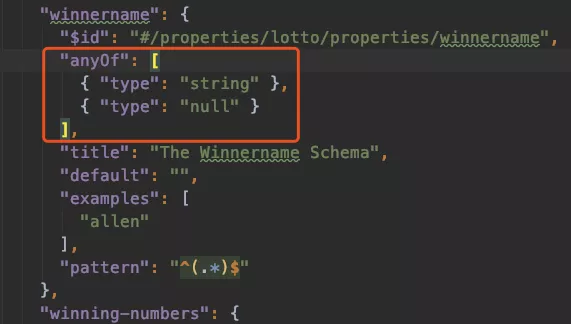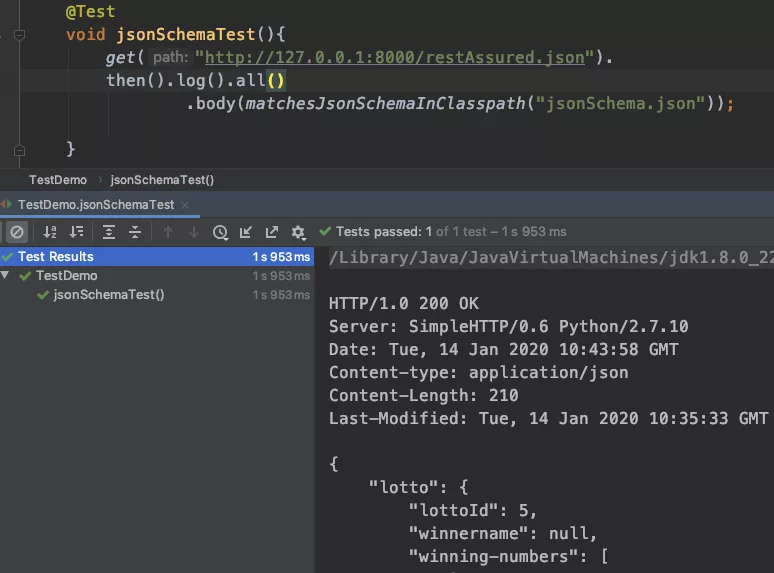# 利器 | REST Assured 实践(二)：断言实现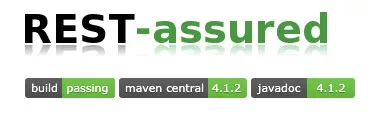`在上一篇文章中，我们初步探讨了 REST Assured 的应用实践，还有很` 多丰富的用法需要慢慢探索研究。而 `REST Assured ` 提供的完整断言手段，是测试工程师最常用最重要的功能之一。断言该如何使用呢？

1. Json 断言

1.1 环境准备

``````{
``````
``````"lotto":{
``````
`````` "lottoId":5,
``````
`````` "winning-numbers":[2,45,34,23,7,5,3],
``````
`````` "winners":[{
``````
``````   "winnerId":23,
``````
``````   "numbers":[2,45,34,23,3,5]
``````
`````` },{
``````
``````   "winnerId":54,
``````
``````   "numbers":[52,3,12,11,18,22]
``````
`````` }]
``````
``````}
``````
``````}
``````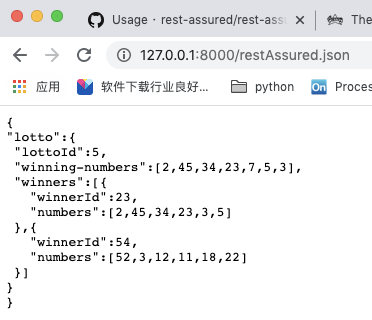1.2 JsonPath(Groovy’s GPath)

1）我们可以使用根节点.(点)子节点的方式一层层的找下去，例如我们需要对 `lottoId` 等于 5 进行断言：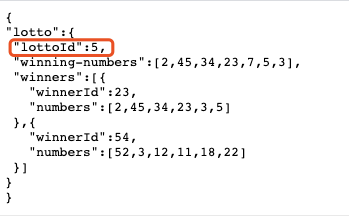``````@Test
``````
``````void testGPath(){
``````
``````     given().
``````
``````     when().
``````
``````             log().all().get("http://127.0.0.1:8000/restAssured.json").
``````
``````     then().
``````
``````             log().all().body("lotto.lottoId",equalTo(5));
``````
`````` }
``````

2）如果我们想要断言 `winners` 数组下面的 `winnerId` ，检查23和54是否包含其中，可以如下 `lotto.winners.winnerId` 写法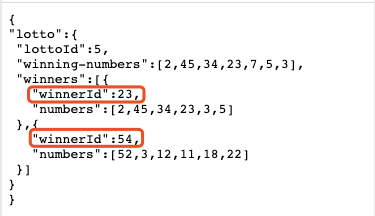``````@Test
``````
``````void testGPath(){
``````
``````    given().
``````
``````    when().
``````
``````            log().all().get("http://127.0.0.1:8000/restAssured.json").
``````
``````    then().
``````
``````            log().all()
``````
``````            .body("lotto.winners.winnerId",hasItems(54,23));
``````
``````}
``````

1）如果我们想要取某些相同字段中的某一个，可以使用类似索引的方式获取，例如想要断言 `winners ` 数组下面的 `winnerId ` 的第一个值是否为23，可以使用 `lotto.winners.winnerId` ，写法如下：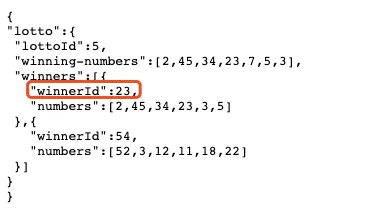``````@Test
``````
``````void testGPath(){
``````
``````    given().
``````
``````    when().
``````
``````            log().all().get("http://127.0.0.1:8000/restAssured.json").
``````
``````    then().
``````
``````            log().all()
``````
``````            .body("lotto.winners.winnerId",equalTo(23));
``````
``````}
``````

2）如果我们想要取某些相同字段中的最后一个，可以使用 `-1 ` 作为索引，例如断言断言 `winners ` 数组下面的 `winnerId ` 的最后一个的值是否为 54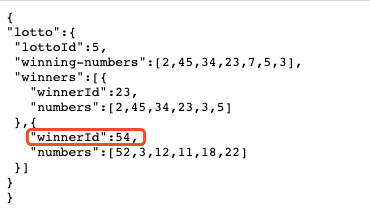``````@Test
``````
``````void testGPath(){
``````
``````    given().
``````
``````    when().
``````
``````            log().all().get("http://127.0.0.1:8000/restAssured.json").
``````
``````    then().
``````
``````            log().all()
``````
``````            .body("lotto.winners.winnerId[-1]",equalTo(54));
``````
``````}
``````

findAll

``````@Test
``````
``````void testGPath(){
``````
``````    given().
``````
``````    when().
``````
``````            log().all().get("http://127.0.0.1:8000/restAssured.json").
``````
``````    then().
``````
``````            log().all()
``````
``````            .body("lotto.winners.findAll{ winners -> winners.winnerId >= 30 && winners.winnerId < 60}.winnerId",equalTo(54));
``````
``````}
``````

find
find 的用法与 findAll 基本一致，只是 find 默认取匹配到的第一个：

``````@Test
``````
``````void testGPath(){
``````
``````    given().
``````
``````    when().
``````
``````            log().all().get("http://127.0.0.1:8000/restAssured.json").
``````
``````    then().
``````
``````            log().all()
``````
``````            .body("lotto.winners.find{ winners -> winners.winnerId >= 30 && winners.winnerId < 60}.winnerId",equalTo(54));
``````
``````    }
``````

1.3 实操演示

2. XML断言

2.1 环境准备

``````<shopping>
``````
`````` <category type="groceries">
``````
`````` <item>
``````
`````` <name>Chocolate</name>
``````
`````` <price>10</price>
``````
`````` </item>
``````
`````` <item>
``````
`````` <name>Coffee</name>
``````
`````` <price>20</price>
``````
`````` </item>
``````
`````` </category>
``````
`````` <category type="supplies">
``````
`````` <item>
``````
`````` <name>Paper</name>
``````
`````` <price>5</price>
``````
`````` </item>
``````
`````` <item quantity="4">
``````
`````` <name>Pens</name>
``````
`````` <price>15</price>
``````
`````` </item>
``````
`````` </category>
``````
`````` <category type="present">
``````
`````` <item when="Aug 10">
``````
`````` <name>Kathryn's Birthday</name>
``````
`````` <price>200</price>
``````
`````` </item>
``````
`````` </category>
``````
`````` </shopping>
``````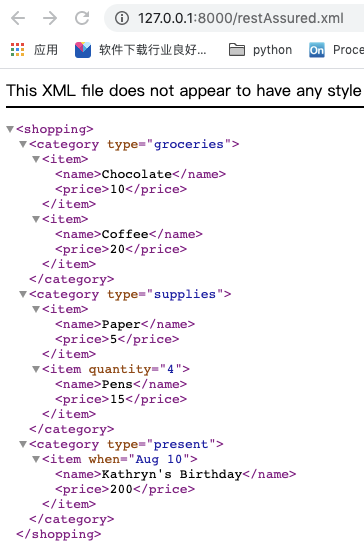2.2 XmlPath断言语法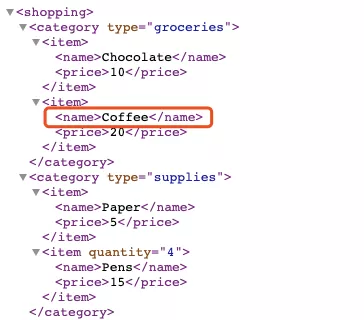``````@Test
``````
``````void testXML(){
``````
``````    when().
``````
``````            get("http://127.0.0.1:8000/restAssured.xml").
``````
``````    then().
``````
``````            log().all().
``````
``````            body("shopping.category.item.name",equalTo("Coffee"));
``````
``````}
``````

size()

``````@Test
``````
``````void testXML(){
``````
``````    when().
``````
``````            get("http://127.0.0.1:8000/restAssured.xml").
``````
``````    then().
``````
``````            log().all()
``````
``````            .body("shopping.category.size()",equalTo(3));
``````
``````}
``````

it.@type、it.price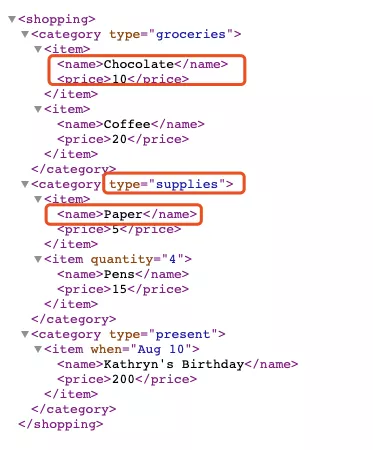``````@Test
``````
``````void testXML(){
``````
``````     when().
``````
``````             get("http://127.0.0.1:8000/restAssured.xml").
``````
``````     then().
``````
``````             log().all()
``````
``````             .body("shopping.category.findAll{ it.@type == 'supplies' }.item.name",equalTo("Paper"))
``````
``````             .body("shopping.category.item.findAll{ it.price == 10 }.name",equalTo("Chocolate"));
``````
`````` }
``````

**.findAll

``````@Test
``````
``````void testXML(){
``````
``````    when().
``````
``````            get("http://127.0.0.1:8000/restAssured.xml").
``````
``````    then().
``````
``````            log().all()
``````
``````            .body("**.findAll{ it.price == 10 }.name",equalTo("Chocolate"));
``````
``````}
``````

2.3 实操演示

3. JsonSchema断言

3.1 需求背景

3.2 使用方法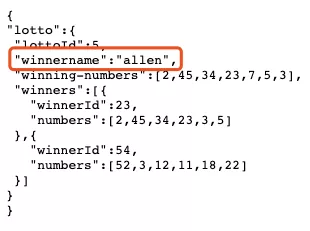##### JsonSchema模板生成

1）首先要借助于 `Json schema tool` 的网站https://www.jsonschema.net/，将返回json字符串复制到页面左边，然后点击 `INFER SHCEMA` ,就会自动转换为 `schema json` 文件类型,会将每个地段的返回值类型都设置一个默认类型; 在 `pattern` 中也可以写正则进行匹配

2）点击 `“设置”` 按钮会出现各个类型返回值更详细的断言设置，这个就是 `schema` 最常用也是最实用的功能，也可以对每种类型的字段最更细化的区间值校验或者断言，例如长度，取值范围等，具体感兴趣的话可以从官网学习深入学习；平常对重要字段的校验我通常会选用其他断言，比如 `hamcrest` 断言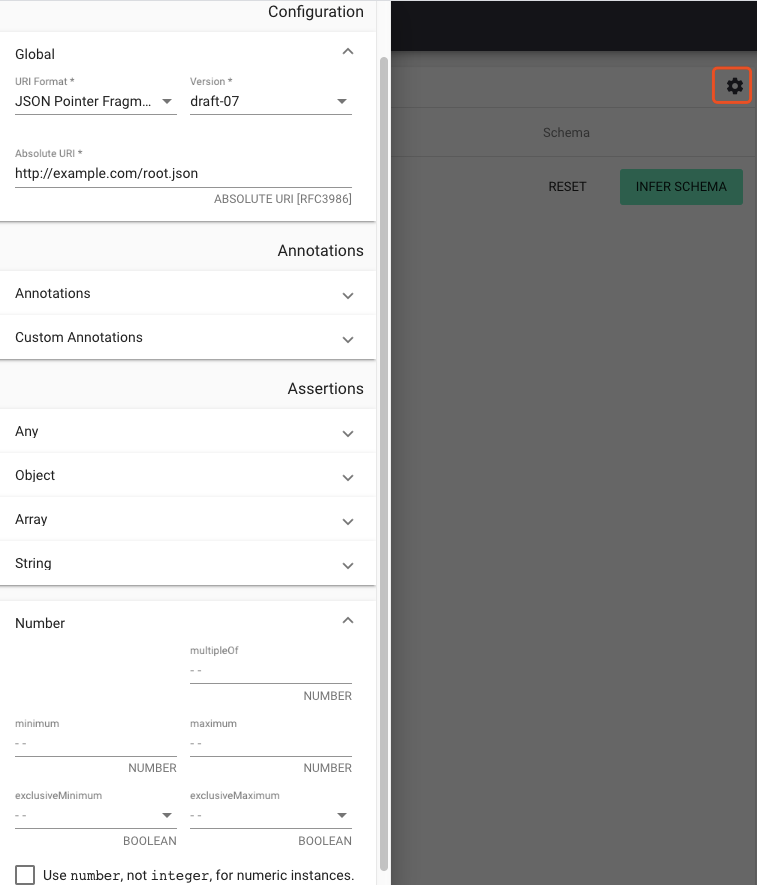3）选择复制功能，可以将生成的 `schema` 模板保存下来

##### rest-assured结合使用

4）添加maven依赖，在 `rest-assured` 完成支持

``````<dependency>
``````
``````    <groupId>io.rest-assured</groupId>
``````
``````    <artifactId>json-schema-validator</artifactId>
``````
``````    <version>4.0.0</version>
``````
``````</dependency>
``````

5）使用 `matchesJsonSchemaInClasspath` 方法对响应结果进行 `schema` 断言

``````@Test
``````
``````void jsonSchemaTest(){
``````
``````    get("http://127.0.0.1:8000/restAssured.json").
``````
``````    then().log().all()
``````
``````            .body(matchesJsonSchemaInClasspath("jsonSchema.json"));
``````
``````}
``````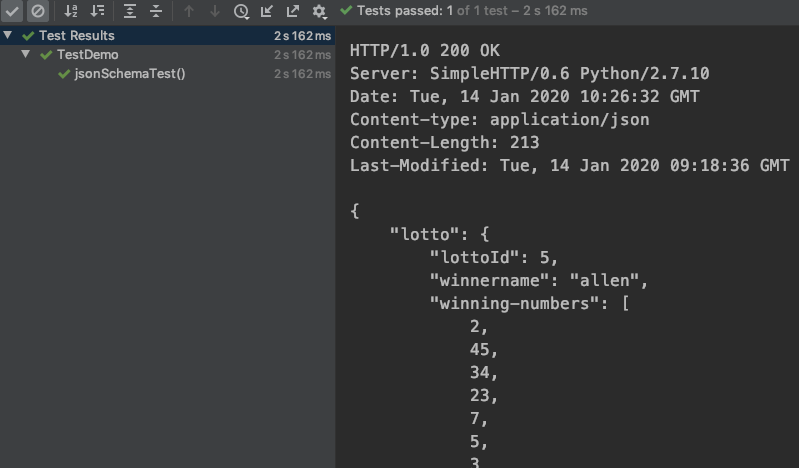##### 多类型校验-Combining schemas
• String类型的默认值为null，后端很有可能在某个字段无值时返回null，例如我们将之前添加的 `winnername` 字段返回 `null` :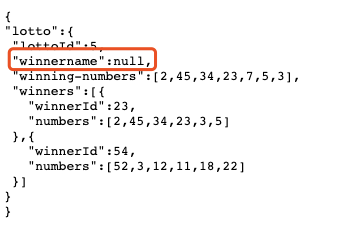• allOf
• anyOf
• oneOf
• not